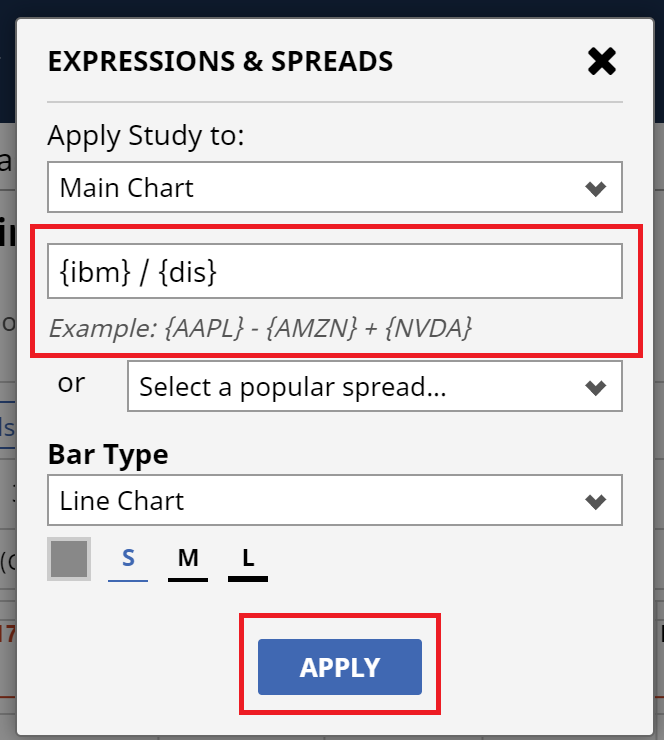You can plot an expression or common futures spread by clicking on the "f(x)" button, or by opening the Chart Menu (the three horizontal bars top right of the chart) and selecting "Expressions & Spreads". You may also right-click on the chart to select the same command.

The Expressions dialog allows you to choose from a number of popular commodity spreads. When you select a popular spread from the drop-down list, the expression is built automatically for you. You may also create your own custom spread chart by entering the mathematical calculation.

The calculated results are displayed using the bar type you choose for the expression. Additionally, an expression can be added to the main chart window, or as a new pane on the chart (you'll choose the placement when you create the expression).

If you want to create your own custom expression, you can enter the calculation directly into the expression field. Expressions consist of:

symbols - must be enclosed in "curly brackets". Examples: {ZC*1} {ESU17} {IBM} {^EURUSD}

constants: any floating point number like 2.35; note that we don't support "scientific" notation (for example 1.72E5)

operators:

unary operator - (negation) so you may enter -2 + 1 and are not forced to type 1 - 2

binary operators:

- (subtraction)

* (multiplication)

/ (division)

^(power)

These are listed in order from lowest to highest precedence (multiplication and division will always happen before addition and subtraction, unless grouped by parentheses).

to make sure precedence is correct, parentheses ( and ) can be used; for example 2 ^ 2 + 3 = 7 but 2 ^ (2 + 3) = 32

An example, showcasing all variables:

{ESM20} * 4.75 - (-(1.5 + {ES*2} / 33) ^ 2 * 4.25)

To invert the scale for a symbol, enter an expression with a zero preceding the symbol: 0-{SPY} or 0-{IBM}

Note for Futures Contracts: Barchart's charting application commonly uses the * symbol on futures contracts as a shortcut to specify the month. For example, ZC*1 will return the front month, ZC*2 returns the second month out, ZC*3 returns the third month out, etc. Using shortcut symbols when building an expression is allowable:

({ZC*1} + {ZC*2} + {ZC*3}) / 3 will add the front, second and third months for Corn, then divide the result by three.f(x) expression box